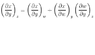# Where did this equation come from?

• pivoxa15
The Attempt at a SolutionThe Attempt at a SolutionIn summary, the equation shown is a mathematical identity that is used in thermal physics. It is derived from a different equation, and requires that the dependent variable, y, be rewritten in terms of w and z.f

## Homework Statement

This equation in the attached document appeared in thermal physics as a mathematical identity. I like to know mathematically how it is derived.

## The Attempt at a Solution

I don't know where to start

#### Attachments

•equation.GIF
3.9 KB · Views: 419
I can't open the gif file. Maybe others can.

A Mentor needs to approve it. They're probably catching up on their Z's, now.That's just the chain rule for partial derivatives- the notation, being physics rather than mathematics is a little peculiar. The subscripts mean "this variable being treated as a constant.

You are right in that the notations are not clear.
I assume that the 4 variables x,y,z,w are dependent variables? But what are the independent variables? Do I need to use the Jacobian?

You are right in that the notations are not clear.
I assume that the 4 variables x,y,z,w are dependent variables? But what are the independent variables? Do I need to use the Jacobian?

On the left side, x is a function of y and z, x(y,z).

Then imagine rewriting this as a function of y and w instead, where w is some function of *both* y and z. The only condition is that the resulting function x(y,w) does not depend on z explicitly (but it does implicitly through the dependence of w on z).

In other words, one goes from x(y,z) to x(y,w(y,z)).

Edit: when I say that w is a function of both y and z, I mean that it *may* be a function of both y and z. Of course, a special and trivial case is w=z. A slightly more general case is w is some function of z. In both cases, obviously the partial derivative of x with respect to y is the same no matter if x is expressed in terms of y,z or in terms of y,w. But if w is a function of both z and y, the formula needed is the one you quoted.

Patrick

Last edited:
I see, how did you work it out?

They should state
if x(y,z)=x(y,w(y,z)) then the equation I showed.